# How to get last word from text string

This post explains that how to extract the last word from a text string in excel. How do I get the last word in a text string using excel formula.

## Extract last word

If you want to get the last word from a text string, you can create an excel formula based on the RIGHT function, the LEN function, the FIND function and the SUBSTITUTE function.

You need to get the position number of the last space character in the text string, so you can use the SUBSTITUTE function in combination with the LEN function to get the length of the text without spaces firstly, then the number is subtracted from the length of the original text to get the number of the space character, then using the SUBSTITUTE function replace the last space character with hash character in the text.

Next, you can use the FIND function to locate the position of the first hash character in the text, it is also the position of the last space character.

Last, the returned position is subtracted by the length of the original text to get the length of the last word, then you can use the RIGHT function to extract it.

Assuming that you want to get the last word from a text string in Cell B1, you can write down the following formula:

`=RIGHT(B1,LEN(B1)-FIND("#", SUBSTITUTE(B1," ","#", LEN(B1)-LEN(SUBSTITUTE(B1," ","")))))`

Let’s see how this formula works:

=LEN(SUBSTITUTE(B1,” “,””)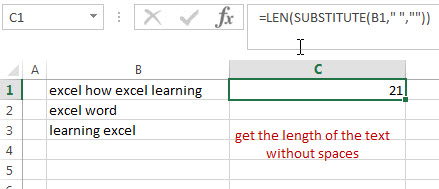The SUBSTITUTE function replaces all space characters with empty character to remove all spaces, and then using LEN function to get the length of the text without spaces.

=LEN(B1)-LEN(SUBSTITUTE(B1,” “,””))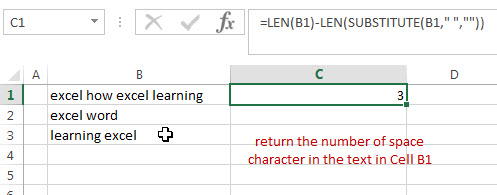This formula will return the number of space character in the text in Cell B1.

=SUBSTITUTE(B1,” “,”#”, LEN(B1)-LEN(SUBSTITUTE(B1,” “,””)))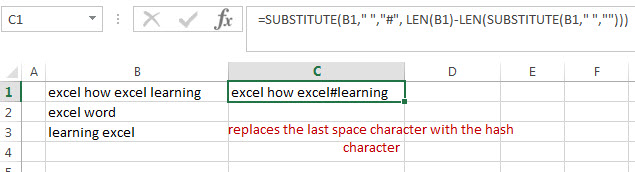This formula replaces the last space character with the hash character.

=FIND(“#”, SUBSTITUTE(B1,” “,”#”, LEN(B1)-LEN(SUBSTITUTE(B1,” “,””))))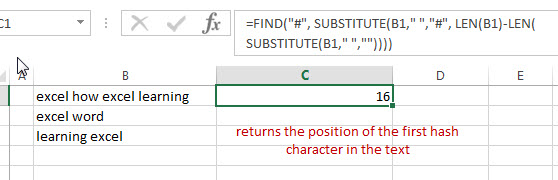This formula returns the position of the first hash character in the text and the number is also the position of the last space character in the text in Cell B1.

=LEN(B1)-FIND(“#”, SUBSTITUTE(B1,” “,”#”, LEN(B1)-LEN(SUBSTITUTE(B1,” “,””))))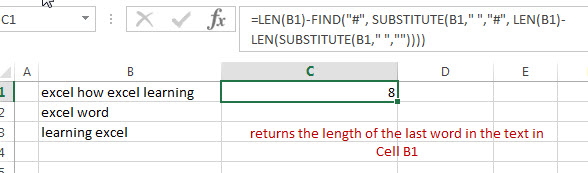This formula returns the length of the last word in the text in Cell B1. It returns 8.

=RIGHT(B1,8)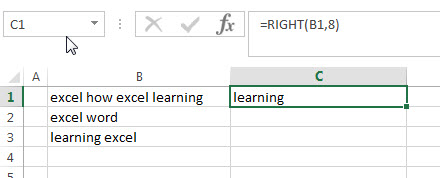This formula extracts the right-most 8 characters and it is also the last word in the text.

### Related Formulas

• Get first word from text string
If you want to extract the first word from a text string in a cell, you can use a combination of the IF function, the ISERR function, the LEFT function and the FIND function to create a complex excel formula..…
• Extract nth word from text string
If you want to extract the nth word from a text string in a single cell, you can create an excel formula based on the TRIM function, the MID function, the SUBSTITUTE function, the REPT function and the LEN function..…
• Split Multiple Lines from a Cell into Rows
If you have multiple lines in a cell and each line is separated by line break character or press “alt + enter” key while you entering the text string into cells, and you need to extract the multiple lines into the separated rows or columns, you can use a combination with the TRIM function, the MID function, the SUBSTITUTE function, the REPT function, the LEN function to create a complex excel formula..…
• count specific words in a cell or a range
If you want to count the number of a specific word in a single cell, you need to use the SUBSTITUTE function to remove all that certain word in text string, then using LEN function to calculate the length of the substring that without that specific word.…
• Extract word that starting with a specific character
Assuming that you have a text string that contains email address in Cell B1, and if you want to extract word that begins with a specific character “@” sign, you can use a combination with the TRIM function, the LEFT function, the SUBSTITUTE function, the MID function, the FIND function, the LEN function and the REPT function to create an excel formula.…

### Related Functions

• Excel RIGHT function
The Excel RIGHT function returns a substring (a specified number of the characters) from a text string, starting from the rightmost character.The syntax of the RIGHT function is as below:= RIGHT (text,[num_chars])…
• Excel Substitute function
The Excel SUBSTITUTE function replaces a new text string for an old text string in a text string.The syntax of the SUBSTITUTE function is as below:= SUBSTITUTE  (text, old_text, new_text,[instance_num])….
• Excel FIND function
The Excel FIND function returns the position of the first text string (sub string) within another text string.The syntax of the FIND function is as below:= FIND(find_text, within_text,[start_num])…
• Excel LEN function
The Excel LEN function returns the length of a text string (the number of characters in a text string).The syntax of the LEN function is as below:= LEN(text)…